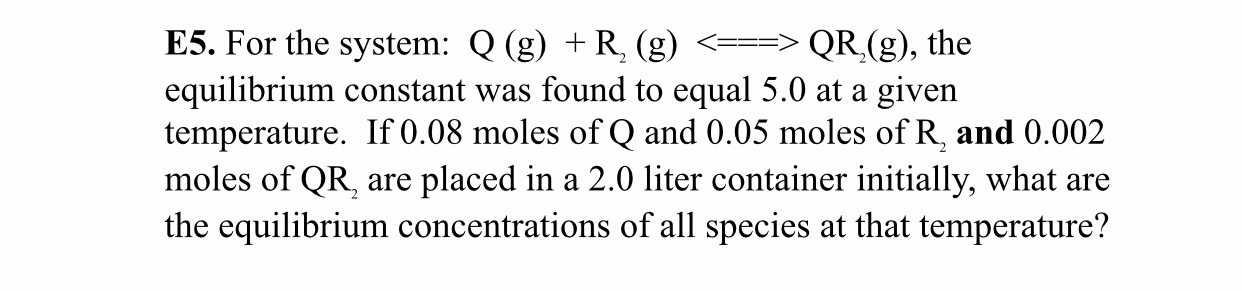# For the system: Q (g) + R2 (g) ⇌ QR2 (g), the equilibrium constant was found to equal 5.0 at a given temperature. If 0.08 moles of Q and 0.05 moles of R2 and 0.002 moles of QR2 are placed ina 2.0 liter container initially, what are the equilibrium concentrations of all species at that temperature?